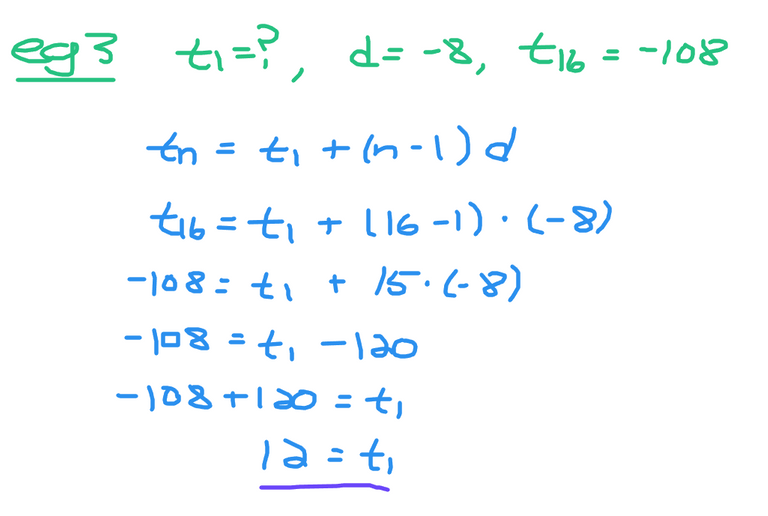# Arithmetic Sequences - A Guide

in STEMGeekslast month

Hi there. In this guide, I cover the math topic of arithmetic sequences.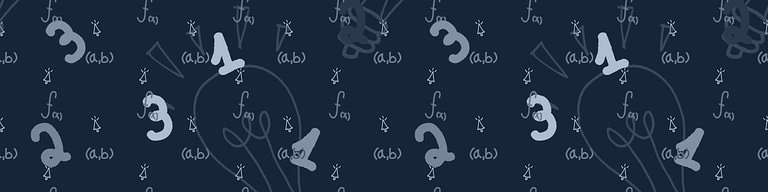Pixabay Image Source

## Topics

• Basic Number Patterns
• The Arithmetic Sequence
• Examples Of Solving For Missing Values In Arithmetic Sequences

## Basic Number Patterns

Before getting into the definition of a arithmetic sequence I would like to share some basic number patterns. That is the number patterns you would have most likely seen as a kid or young student.

Count By 2

`2, 4, 6, 8, 10, 12, ..., 100`

Count By 10 Starting at 7

`7, 17, 27, 37, 47, ..., 177`

Subtract 5 Every Time

`28, 23, 18, 13, 8, 3, -2, -7, -12, ..., -42`

## The Arithmetic Sequence

In a more mathematical setting the arithmetic sequence is based on these basic number patterns where the increase or decrease is the same in getting the next number. Here is a formula for obtaining any number in an arithmetic sequence.

`t_n = t_1 + (n - 1)d`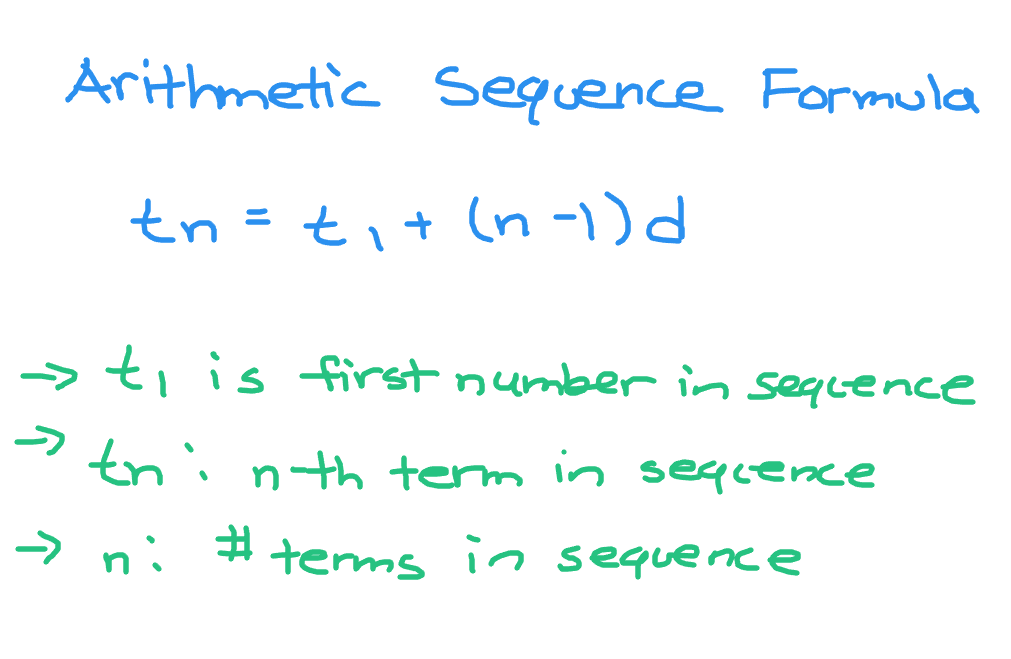Note that the term `t_1` is the first number in the sequence.

The term is `t_n` is the n-th term in the sequence. If `n = 10` we have the tenth number or term in the arithmetic sequence.

The variable `n` is the number of terms in the arithmetic sequence.

The letter `d` represents how much the numbers go up or down in every step. A value of 2 for d would be counting up by 2 to get the next number as an example. A value of `-10` for `d` would be subtracting 10 to get the next number.

Example One - Count By 8 Starting at 2

`2, 10, 18, 26, 34, 42, 50, 58, 66`

The first term here is two with a `d` value of eight. Fifty would be the seventh term in this sequence which you can represent as `t_7 = 50`.

As the number of terms in this sequence is 9 the value for `n` would be 9.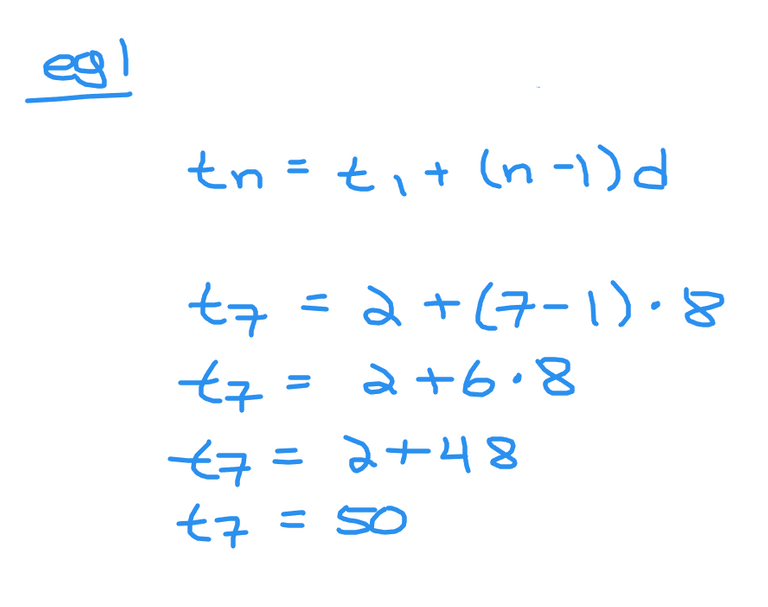Example Two - Subtract By 10 Starting at 59

`59, 49, 39, 29, 19, 9, -1, -11, -21, -31`

With this arithmetic sequence we have:

• Ten values which would make `n = 10`
• The first term as 59
• The final or tenth term as -31
• The common difference as negative ten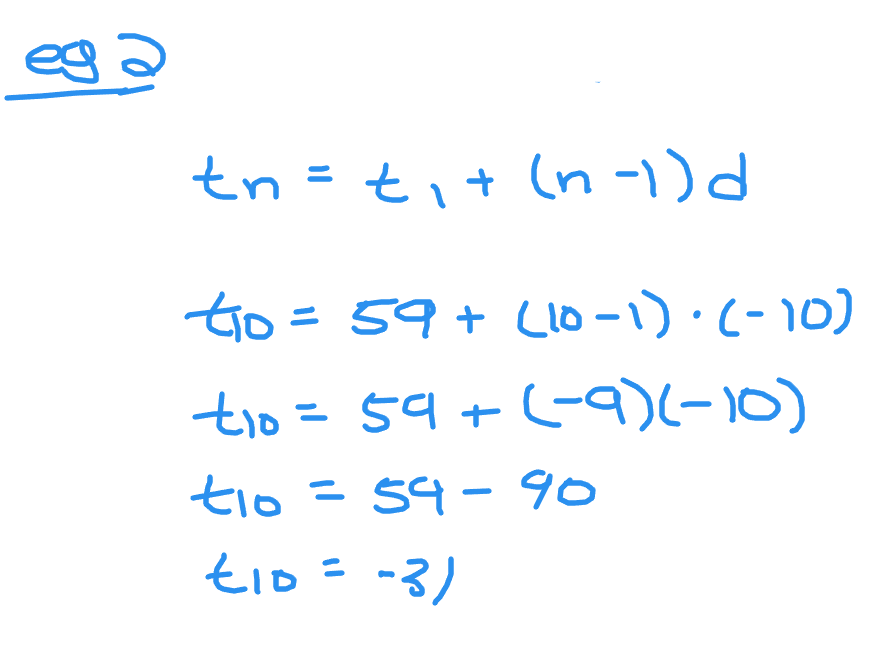## Examples Of Solving For Missing Values In Arithmetic Sequences

The arithmetic sequence formula can be used to find certain numbers in a sequence, the first number in the sequence, how many numbers are there in the sequence or the common difference.

Example One

The first number in the sequence is 2 with a common difference of 5. What is the twentieth number in this arithmetic sequence?

You could start at 2 and count by five until you get number/term 20. That could take a bit of time. The mathematical way of doing this would be using the arithmetic sequence formula.Example Two - Find Number of Terms

Start with the number one and the last number in the arithmetic sequence as 232. The common difference is `11`. How many terms are there in this sequence?

The first number or `t_1` is 1 and let `t_n = 232`. Eleven would be the common difference as in `d = 11`. Use the arithmetic sequence formula, substitute the known values and solve for `n`.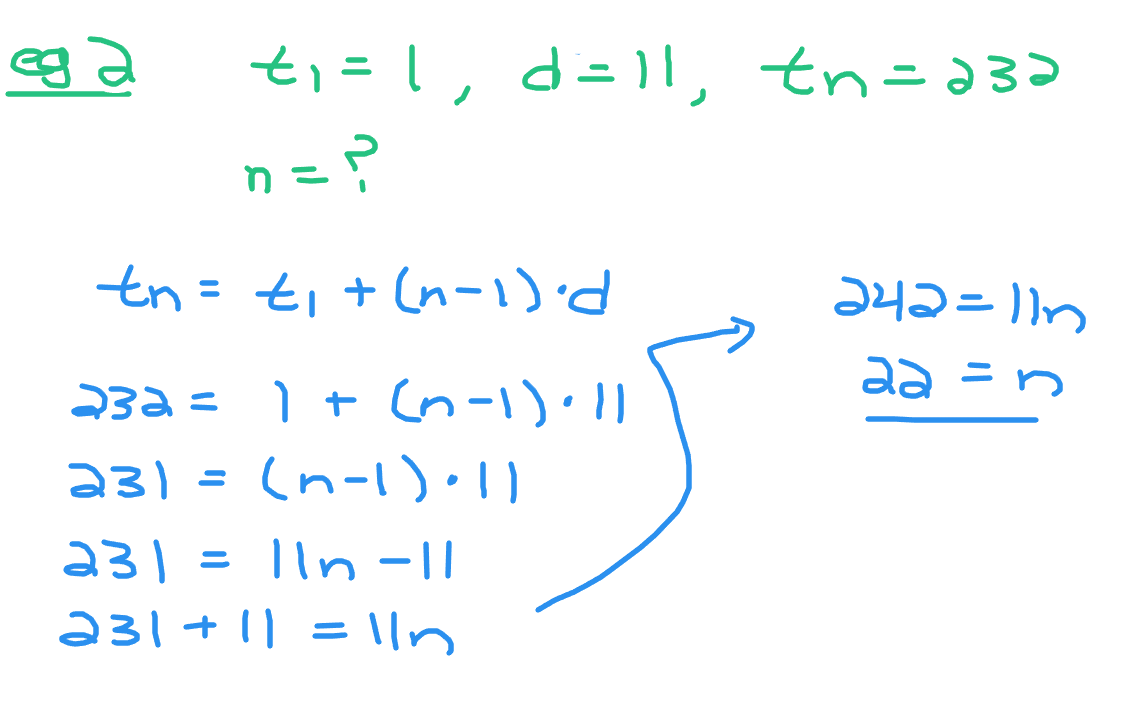Example Three - Determine Starting Number

An arithmetic sequence has a final number of `-108` with a common difference of `-8`. There are 16 numbers in this sequence. What is the first number here?